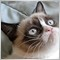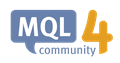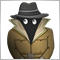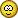963

Hello! I need a quick help with EMA calculation. I seem to be very close, however its incorrect around the 5th decimal place in EURUSD 1M. Any ideas? What's with this? Looks like something with the normalizing? Heres the EA that calculates the EA attached. Can you provide the correct way to have it display the exact same as the normal MT4 EMA as per the code inside EA?
```   period=12;
alpha=2/(period+1);
ema12_default=iMA(_Symbol,PERIOD_M1,12,0,MODE_EMA,PRICE_CLOSE,0);
ema11=iMA(_Symbol,PERIOD_M1,11,0,MODE_EMA,PRICE_CLOSE,0);
close1=iClose(_Symbol,PERIOD_CURRENT,1);
ema12_calculation=ema11+alpha*(close1-ema11);

Print("ema12_calculation = ",ema12_calculation);
Print("ema12_default = ",ema12_default);```
As you can see, I'm using an adapted version of
`EMA = EMA(i-1) + alpha * [Close(1) - EMA(i-1)];`
as per https://www.mql5.com/en/forum/156804.

The version from that post seems be set up to work with arrays. Since I just simply need the value of the current 12 Period EMA, I have adapted it to
`ema11+alpha*(close1-ema11)`
What's keeping it from being exactly the same as the default MT4's EMA value at the 5th decimal?

Free points for the broker.20210

nadiawicket: I need a quick help with EMA calculation. I seem to be very close, however its incorrect around the 5th decimal place in EURUSD 1M. Any ideas?

EMA requires infinite values to be exact.

All depend on how you initially seed the first value.

1. Most make Ema1 equal to Price1.
2. I think MQL4 makes is equal to SMA(l,Bars-l).
3. You are using zero for Ema0
4. I use period=1 .. length for the first length bars.963

whroeder1:

EMA requires infinite values to be exact.

All depend on how you initially seed the first value.

1. Most make Ema1 equal to Price1.
2. I think MQL4 makes is equal to SMA(l,Bars-l).
3. You are using zero for Ema0
4. I use period=1 .. length for the first length bars.

Awesome, Ill check all of this out.

Is there even a way to generate a Price to EMA converter in MQL4? Seems they all require an array of iCloses. So just put the first buffer of the array with the new Bid+1 or whatever custom value I want to use in able to see what the EMA will look like in the first spot of Custom Input Data Array ? And the rest icloses are the same es for regular bid and try imputting those Buffers into the source code?

Making the Bid + 1 (custom value that I want to predict what the EMA would be if bid was at this price) the first of the new array used for the extra digits in the EMA? Just normal Bid does not generate so many digits as using the array as if its more accuracy like that.963

Konstantin Nikitin:
CopyClose

iMA

Just an example. As you need, write.

OK thanks963

```//+------------------------------------------------------------------+
//|                                                          EMA.mq4 |
//|                                                 b-market@mail.ru |
//+------------------------------------------------------------------+

#property indicator_chart_window
#property indicator_buffers 1

double MA_Period12=12;
int MA_Shift12 = 0;
int SetPrice12 = 0;
double ExtMapBuffer12[];
double ema12;
int ExtCountedBars12=0;
double ema12_calculation;
//+------------------------------------------------------------------+
int init()
{
IndicatorDigits(MarketInfo(Symbol(),MODE_DIGITS));
SetIndexShift(0,MA_Shift12);
SetIndexBuffer(0,ExtMapBuffer12);
}
//+------------------------------------------------------------------+
int start()
{
if(Bars <= MA_Period12) return(0);
ExtCountedBars12=IndicatorCounted();
if(ExtCountedBars12 < 0) return(-1);
if(ExtCountedBars12>0) ExtCountedBars12--;
//----
ema12_function();
double pr12;
pr12=2.0/(MA_Period12+1);
int pos12=Bars-2;
if(ExtCountedBars12>2) pos12=Bars-ExtCountedBars12-1;
//---- main calculation loop
while(pos12>=0)
{
if(pos12==Bars-2)
ExtMapBuffer12[pos12+1]=(Open[pos12+1]+Close[pos12+1])/2;
ExtMapBuffer12[pos12]=GetPrice12(pos12)*pr12+ExtMapBuffer12[pos12+1]*(1-pr12);
pos12--;
ema12_calculation=(ExtMapBuffer12[pos12]);
ema12=NormalizeDouble(ema12_calculation,Digits);
Print("ema12 = ",ema12);
return(0);
}
}
//----
void ema12_function()
{
double pr12;
pr12=2.0/(MA_Period12+1);
int pos12=Bars-2;
if(ExtCountedBars12>2) pos12=Bars-ExtCountedBars12-1;
//---- main calculation loop
while(pos12>=0)
{
if(pos12==Bars-2)
ExtMapBuffer12[pos12+1]=(Open[pos12+1]+Close[pos12+1])/2;
ExtMapBuffer12[pos12]=GetPrice12(pos12)*pr12+ExtMapBuffer12[pos12+1]*(1-pr12);
pos12--;
}
}
//+------------------------------------------------------------------+
double GetPrice12(int shift12)
{
double price12;
price12=Close[shift12];
return(price12);
}
//+------------------------------------------------------------------+
```

How do I get to be able to put the price I want so that I know what the EMA is going to be at a certain price? A lot harder than I thought.

Need it to be a Price to EMA converter so I can calculate at what price is the MACD going to be at 0 for my 0 line macd crossing EA. This way I can put a pending order when conditions are met so I get all the points instead of dealing with regular orders which can be late by some points.

Any more ideas/insights?

Tried putting Bid price where

`GetPrice12(pos12)`
goes, since they are both the exact same values. This works perfectly for a while, then it starts messing up. Any ideas why? How do I do this Price to EMA correctly? Has to be a way.963

Konstantin Nikitin:
CopyClose

iMA

Just an example. As you need, write.

How do I give that my custom input bid +1 bid +2 etc?963

whroeder1:

EMA requires infinite values to be exact.

All depend on how you initially seed the first value.

1. Most make Ema1 equal to Price1.
2. I think MQL4 makes is equal to SMA(l,Bars-l).
3. You are using zero for Ema0
4. I use period=1 .. length for the first length ba

Essentially I just need to regenerate the normal EMA array and run it through the function after "customizing" the first close.

So basically just add the "customized" first value into the first close of the array using the buffers as described in https://book.mql4.com/samples/icustomCreation of Custom Indicators - Simple Programs in MQL4 - MQL4 Tutorial
• book.mql4.com
When creating a trading strategy a developer often faces the necessity to draw graphically in a security window a certain dependence calculated by a user (programmer). For this purpose MQL4 offers the possibility of creating custom indicators. Custom Indicator is an application program coded in MQL4; it is basically intended for graphical...963

`ExtMapBuffer12[pos12]=GetPrice12(pos12)*pr12+ExtMapBuffer12[pos12+1]*(1-pr12);`
How to alter the previous line from source to give me bid +1*Digit?963

Konstantin Nikitin:
CopyClose

iMA

Just an example. As you need, write.

```//+------------------------------------------------------------------+
//|                                                     forumema.mq4 |
//|                        Copyright 2017, MetaQuotes Software Corp. |
//|                                             https://www.mql5.com |
//+------------------------------------------------------------------+
#property version   "1.00"
#property strict
#property indicator_chart_window

int       handle;
double    ema12_default, ema11,
close1, _close1[],
iMA_buffer[],  iMA_buffer1[];
//+------------------------------------------------------------------+
//| Custom indicator initialization function                         |
//+------------------------------------------------------------------+
int OnInit()
{
//--- indicator buffers mapping
handle=iMA(_Symbol,PERIOD_M1,12,0,MODE_EMA,PRICE_CLOSE,0);
handle=iMA(_Symbol,PERIOD_M1,11,0,MODE_EMA,PRICE_CLOSE,0);
ArraySetAsSeries(iMA_buffer,   true);
ArraySetAsSeries(iMA_buffer1,  true);
ArraySetAsSeries(_close1,      true);

//---
return(INIT_SUCCEEDED);
}
//+------------------------------------------------------------------+
//| Custom indicator iteration function                              |
//+------------------------------------------------------------------+
void OnTick()
{
if(CopyBuffer(handle,0,0,1,iMA_buffer)==1)
ema12_default = iMA_buffer;
if(CopyBuffer(handle,0,0,1,iMA_buffer1)==1)
ema11 = iMA_buffer1;

if( CopyClose( _Symbol, PERIOD_CURRENT, 1, 1, _close1) == 1 )
close1 = _close1;
}

//---

//--- return value of prev_calculated for next call

//+------------------------------------------------------------------+
```

'Copy Buffer' - function not defined on compile . .

II dont really understand what you are trying to get at with this code.7863

'Copy Buffer' - function not defined on compile . .

II dont really understand what you are trying to get at with this code.

Didn't notice that the topic MQL4963

Konstantin Nikitin:

Didn't notice that the topic MQL4

ok thx anyway